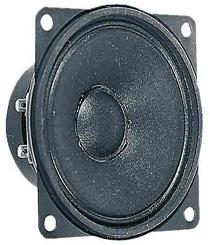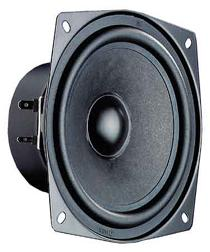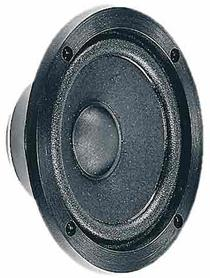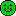Tweeter Mids Woofer Fullrange Coaxial speaker Mini Exciter Horn Driver in Wall ELA no longer available

Visaton

With 40 years of experience in the field of acoustics and loudspeaker technology Visaton is a future oriented Company. With high engagement in research and development plus the high quality standards of production, Visaton is a specialised and world wide successfully operating German loudspeaker manufacturer.

MidsM10-8       order no. V-9089       EUR 12,90excl. VAT: € 10.84 / \$ 12.14
10 cm midrange driver with high quality cone.
• power handling (continuous/programme) = 80/100 W
• frequency range = 450-13000 Hz
• resonance frequency fs = 700 Hz
• impedance R = 8 Ohm
• sound pressure level SPL = 90 dB (2,83V; 1m)
• voice coil diameter = 20 mm
• mounting diameter d = 95 mm
• overall diameter d = 117 mm
• mounting depth (not countersunk) t = 39 mmSC13       order no. V-8013       EUR 26,90excl. VAT: € 22.61 / \$ 25.32
12 cm midrange driver with high quality conet.
• power handling (continuous/programme) = 40/60 W
• frequency range = 90-13000 Hz
• resonance frequency fs = 75 Hz
• impedance R = 8 Ohm
• sound pressure level SPL = 90 dB (2,83V; 1m)
• DC resistance Re = 7 Ohm
• force factor BL = 4,2 N/A
• voice coil inductance L = 0,66 mH
• effective piston radiating area Sd = 79 cm2
• effective mechanical mass incl. air load mms = 4,8 g
• equivalent volume of compliance Vas = 8 l
• total Q factor Qts = 0,73 (Qms=3,73, Qes=0,9)
• voice coil diameter = 20 mm
• maximum peak linear excursion vibration xlin = +/- 0,75 mm
• mounting diameter d = 113 mm
• overall diameter d = 131 mm
• mounting depth (not countersunk) t = 61 mmWS13E       order no. V-1053       EUR 16,90excl. VAT: € 14.20 / \$ 15.91
12 cm midrange driver with paper conet.
• power handling (continuous/programme) = 40/60 W
• frequency range = 90-12000 Hz
• resonance frequency fs = 83 Hz
• impedance R = 8 Ohm
• sound pressure level SPL = 86 dB (2,83V; 1m)
• DC resistance Re = 7,7 Ohm
• force factor BL = 3,6 N/A
• voice coil inductance L = 0,75 mH
• effective piston radiating area Sd = 86,5 cm2
• effective mechanical mass incl. air load mms = 5,1 g
• equivalent volume of compliance Vas = 7,7 l
• total Q factor Qts = 1,08 (Qms=3,52, Qes=1,56)
• voice coil diameter = 20 mm
• maximum peak linear excursion vibration xlin = +/- 0,8 mm
• mounting diameter d = 113 mm
• overall diameter d = 131 mm
• mounting depth (not countersunk) t = 51 mmMR130       order no. V-9016       EUR 19,90excl. VAT: € 16.72 / \$ 18.73
12 cm midrange driver with high quality cone.
• power handling (continuous/programme) = 80/100 W
• frequency range = 400-13000 Hz
• resonance frequency fs = 500 Hz
• impedance R = 8 Ohm
• sound pressure level SPL = 89 dB (2,83V; 1m)
• DC resistance Re = 6,8 Ohm
• force factor BL = 4,3 N/A
• voice coil inductance L = 0,42 mH
• effective piston radiating area Sd = 70 cm2
• effective mechanical mass incl. air load mms = 5 g
• equivalent volume of compliance Vas = 0,14 l
• total Q factor Qts = 3,49 (Qms=8,67, Qes=5,85)
• voice coil diameter = 25 mm
• mounting diameter d = 110 mm
• overall diameter d = 136 mm
• mounting depth (not countersunk) t = 39 mmrecommended cabinet 1:
closed cabinet with 2,8 L volume
from 106/67 Hz (-3dB/-8dB)

recommended cabinet 2:
10,1 L volume bass reflex cabinet
with HP50 reflex tube, 15 cm long.
from 53/43 Hz (-3dB/-8dB).

AL 130 M 8 Ohm       order no. V-1305       EUR 99,00excl. VAT: € 83.19 / \$ 93.18
14 cm midrange driver with high quality cone .
• power handling (continuous/programme) = 150/200 W
• frequency range = 60-8000 Hz
• resonance frequency fs = 50 Hz
• impedance R = 8 Ohm
• sound pressure level SPL = 90 dB (2,83V; 1m)
• DC resistance Re = 5,4 Ohm
• force factor BL = 5,58 N/A
• voice coil inductance L = 0,75 mH
• effective piston radiating area Sd = 78,5 cm2
• effective mechanical mass incl. air load mms = 7,6 g
• equivalent volume of compliance Vas = 11,6 l
• total Q factor Qts = 0,38 (Qms=5,38, Qes=0,41)
• voice coil diameter = 25 mm
• maximum peak linear excursion vibration xlin = +/- 3 mm
• mounting diameter d = 114 mm
• overall diameter d = 153 mm
• mounting depth (not countersunk) t = 58 mmrecommended cabinet 1:
closed cabinet with 5,7 L volume
from 92/58 Hz (-3dB/-8dB)

recommended cabinet 2:
18 L volume bass reflex cabinet
with HP70 reflex tube, 22 cm long.
from 49/38 Hz (-3dB/-8dB).

AL 170 8 Ohm       order no. V-1302       EUR 109,00excl. VAT: € 91.60 / \$ 102.59
16 cm midrange driver with high quality conet.
• power handling (continuous/programme) = 70/100 W
• frequency range = 45-10000 Hz
• resonance frequency fs = 38 Hz
• impedance R = 8 Ohm
• sound pressure level SPL = 88 dB (2,83V; 1m)
• DC resistance Re = 5,6 Ohm
• force factor BL = 6,93 N/A
• voice coil inductance L = 0,91 mH
• effective piston radiating area Sd = 133 cm2
• effective mechanical mass incl. air load mms = 13 g
• equivalent volume of compliance Vas = 33 l
• total Q factor Qts = 0,33 (Qms=4,47, Qes=0,36)
• voice coil diameter = 25 mm
• maximum peak linear excursion vibration xlin = +/- 6 mm
• mounting diameter d = 148 mm
• overall diameter d = 180 mm
• mounting depth (not countersunk) t = 63 mm

 Tweeter Mids Woofer Fullrange Coaxial speaker Mini Exciter Horn Driver in Wall ELA no longer available
The cabinet volume calculations are executed considering an external resistance of 0.3 Ohm. We are using, if no other data is known or available, the information supplied by the manufacturer.

Please note: the frequency response doesn't say much about the cut-off frequency inside the cabinet! Therefore, we mostly give you 2 examples with dimensioning considerations and an indication, how low the loudspeaker will reproduce.

Home